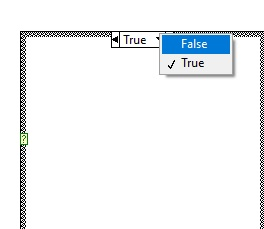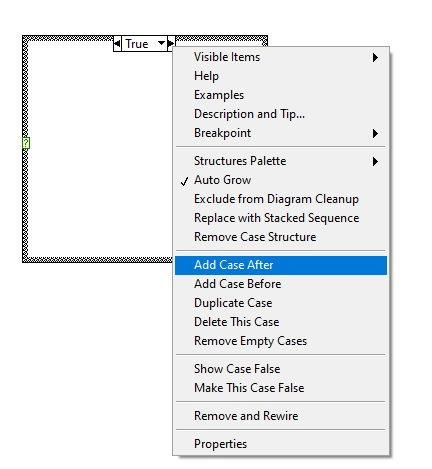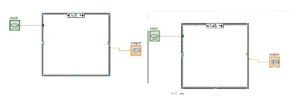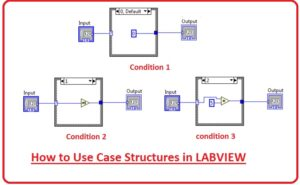Hello, readers welcome to the post. Here we will discuss How to Use Case Structures in LABVIEW. Case structure is a function that is used to implement the selective part of code designed for any project. In other words, it helps to apply the single portion of projects according to the requirements of projects. It helps to apply one part out of numerous and more than one according to needs.

It is considered a branch control process that is applied for numerous functions according to assigned parameters.  I hope you are familiar to the case block used in C++ case structure is like that. In this post, we will discuss it in detail and make a project. To have a detailed understanding. So let get started.

## How to Use Case Structures in LABVIEW

• To use case structure move to block diagram then press right go to structure and select the case structure option and place it in block diagram• Look at the case structure can seen here.• if you move your cursor to the left corner of structure then a yellow box is seen move your cursor at that will see the case selector mentioned tha is the input of the case structure.
• The case selector helpful for us to tell that which case will be applied according to given below statements
• Let us suppose that we have three components X, Y, Z and the value case selector is A
• if(A == X) case work for X
• if(A == Y) case work for Y
• if(A == Z) case work for Z
• There is undefined value for the case selector so before using it must have required data to the case selector.
• At top of the case structure there is a box exit whre the values of our function is placed according to requirements.• In this box the case values are selected every case has it own component.
• When we have an assing case structure we need to place cases at the case structure.
• for this process press right at the case structure and choose to add case before or add case after according to the desired state. can see here.• These cases will help us to implement function through case structure application.
• Let us take an example and see that the output of the case structure will be one if its output value is true and output will be zero if input is false.• For practical understating, we take an example. In the below figure you can see that three conditions are satisfied.
• The output of our project will be two if the input is 1 and output is 10 if the input is 2 and output will be 0 if other conditions exist.
• Here we are making the project through the use of the multiplication function.that is all about the How to Use Case Structures in LABVIEW. I have explained each and every parameter related to this post. If you have any further query ask in the comments. Thanks for reading have a nice day